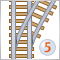Custom indicator MA drawing arrows - Multi currency839

Hi,

I have a custom indicator that calculates a MA line (like ma in MT5 indicator), I plot up arrow and down arrow for the direction. I have a problem when I put this indicator on more than one chart (different currency...it seems they are effecting each other. the last loaded chart doesn't draw arrows while the first draw. What can be wrong?

#property indicator_chart_window
#include <MovingAverages.mqh>
//--- input parameters
input int MAPeriod = 23; //Moving Average Period
int MAShift;
input double DisiredAngle = 0; //Desired Angle
ENUM_MA_METHOD MAMethod = MODE_SMA;
ENUM_APPLIED_PRICE MAAppliedPrice = PRICE_CLOSE;
#property indicator_buffers 3
#property indicator_plots   3

#property indicator_color1 Green
#property indicator_type1  DRAW_ARROW

#property indicator_color2 Red
#property indicator_type2  DRAW_ARROW

#property indicator_color3 Purple
#property indicator_type3  DRAW_LINE

double UpArrow[],DownArrow[],MABuffer[];

int LastArrowIndex;
//+------------------------------------------------------------------+
//| Custom indicator initialization function                         |
//+------------------------------------------------------------------+
int OnInit()
{
//--- indicator buffers mapping
SetIndexBuffer(0, UpArrow, INDICATOR_DATA);
SetIndexBuffer(1, DownArrow, INDICATOR_DATA);
SetIndexBuffer(2, MABuffer, INDICATOR_DATA);
//----
//Setting the Buffer 0 to draw up arrow
PlotIndexSetInteger(0,PLOT_ARROW,233);
PlotIndexSetDouble(0,PLOT_EMPTY_VALUE,0);

//Setting the Buffer 0 to draw down arrow
PlotIndexSetInteger(1,PLOT_ARROW,234);
PlotIndexSetDouble(1,PLOT_EMPTY_VALUE,0);

PlotIndexSetDouble(2,PLOT_EMPTY_VALUE,0);
//----
IndicatorSetString(INDICATOR_SHORTNAME,"Moving Average (" + MAPeriod +","+DisiredAngle+") ");
//---
return(INIT_SUCCEEDED);
}
//+------------------------------------------------------------------+
//| Custom indicator iteration function                              |
//+------------------------------------------------------------------+
int OnCalculate(const int rates_total,
const int prev_calculated,
const int begin,
const double &price[])
{

SimpleMAOnBuffer(rates_total,0,begin,MAPeriod,price,MABuffer );
//--- check for bars count
if(rates_total<MAPeriod-1+begin)
return(0);// not enough bars for calculation

//--- first time we run indicator
if(prev_calculated==0)
{
ArrayInitialize(UpArrow,0);
ArrayInitialize(DownArrow,0);
}

//How many new bars are there for this call
for(int i = rates_total-1; (i >= (rates_total - prev_calculated))&&(i>2); i--)
{
//up arrow logic - (curr value - prev timeframe value) is > (prev timeframe value - prev - 1 timeframe value)

//If the diffrence between the latest bar and 1 previous has reach the desired diffrence (Angle) then return true. Or if DesiredAngle is disable <=0.
bool HasReachDisiredAngle = ((MABuffer[i]-MABuffer[i-1]) > DisiredAngle)||(DisiredAngle<=0)?true:false;
if(
(MABuffer[i-1]>MABuffer[i-2]) &&
(MABuffer[i] > MABuffer[i-1]) &&
((MABuffer[i-1]-MABuffer[i-2]) > 0) &&
((MABuffer[i]-MABuffer[i-1])>0) &&
HasReachDisiredAngle
)
{
UpArrow[i] = MABuffer[i];
}

HasReachDisiredAngle = ((MABuffer[i-1]-MABuffer[i]) > DisiredAngle)||(DisiredAngle<=0)?true:false;
if(
(MABuffer[i-1]<MABuffer[i-2]) &&
(MABuffer[i] < MABuffer[i-1]) &&
((MABuffer[i-1]-MABuffer[i-2]) < 0) &&
((MABuffer[i]-MABuffer[i-1])<0) &&
HasReachDisiredAngle
)
{
DownArrow[i] = MABuffer[i];
}

}

//--- return value of prev_calculated for next call
return(rates_total);
}Step on New Rails: Custom Indicators in MQL5
• 2009.11.23
• Андрей
• www.mql5.com
I will not list all of the new possibilities and features of the new terminal and language. They are numerous, and some novelties are worth the discussion in a separate article. Also there is no code here, written with object-oriented programming, it is a too serous topic to be simply mentioned in a context as additional advantages for developers. In this article we will consider the indicators, their structure, drawing, types and their programming details, as compared to MQL4. I hope that this article will be useful both for beginners and experienced developers, maybe some of them will find something new.17734

ToolMaker:

Hi,

I have a custom indicator that calculates a MA line (like ma in MT5 indicator), I plot up arrow and down arrow for the direction. I have a problem when I put this indicator on more than one chart (different currency...it seems they are effecting each other. the last loaded chart doesn't draw arrows while the first draw. What can be wrong?

When you run these Indicators how much CPU usage is the MT5 terminal using ?  how many cores does your CPU have ?  does it have hyperthreading enabled ?839

RaptorUK:
When you run these Indicators how much CPU usage is the MT5 terminal using ?  how many cores does your CPU have ?  does it have hyperthreading enabled ?

Thanks Raptor for your time.

I have i7 8 cores. The indicator is slow at first run (2-3 seconds loading time) but after that all is fine. It also depends on how much data you have PRE-downloaded. if you go delete you history then it would be quick. I can add a counter parameter to be able to specify the amount of periods to calculate but I haven't find the need for it.

I have found my issue, I do a array initialize on start but all new items after initialize dont get set to 0. So I replaced array initialize to just setting the buffers to zero. Thanks again for your time.

int OnCalculate(const int rates_total,
const int prev_calculated,
const int begin,
const double &price[])
{

SimpleMAOnBuffer(rates_total,0,begin,MAPeriod,price,MABuffer );
//--- check for bars count
if(rates_total<MAPeriod-1+begin)
return(0);// not enough bars for calculation

//How many new bars are there for this call
for(int i = rates_total-1; (i >= (rates_total - prev_calculated))&&(i>2); i--)
{
//up arrow logic - (curr value - prev timeframe value) is > (prev timeframe value - prev - 1 timeframe value)
UpArrow[i] = 0;
DownArrow[i] = 0;
//If the diffrence between the latest bar and 1 previous has reach the desired diffrence (Angle) then return true. Or if DesiredAngle is disable <=0.
bool HasReachDisiredAngle = ((MABuffer[i]-MABuffer[i-1]) > DisiredAngle)||(DisiredAngle<=0)?true:false;
if(
(MABuffer[i-1]>MABuffer[i-2]) && ....blah blah